## Paraboloids

 ELLIPTIC PARABOLOID
 elliptic paraboloid  $$\frac{x^2}{a^2}+\frac{y^2}{b^2}=2cz$$ Real plane intersections: the ellipses, the circles and the parabolas. paraboloid of revolution $$\frac{x^2}{a^2}+\frac{y^2}{a^2}=2cz$$ It is obtained by rotating a parabola about its axis. Real plane intersections: the ellipses, the circles and the parabolas.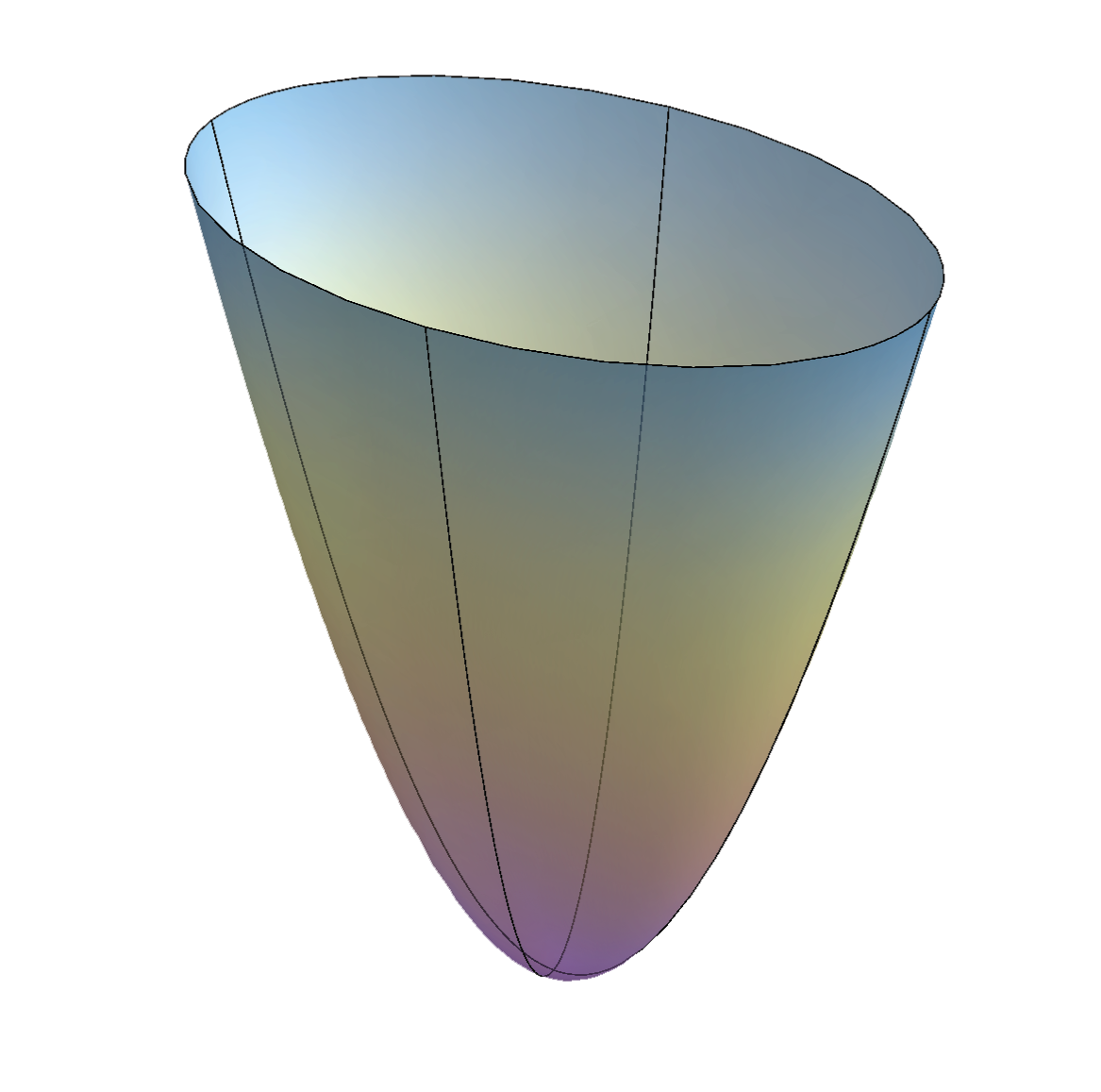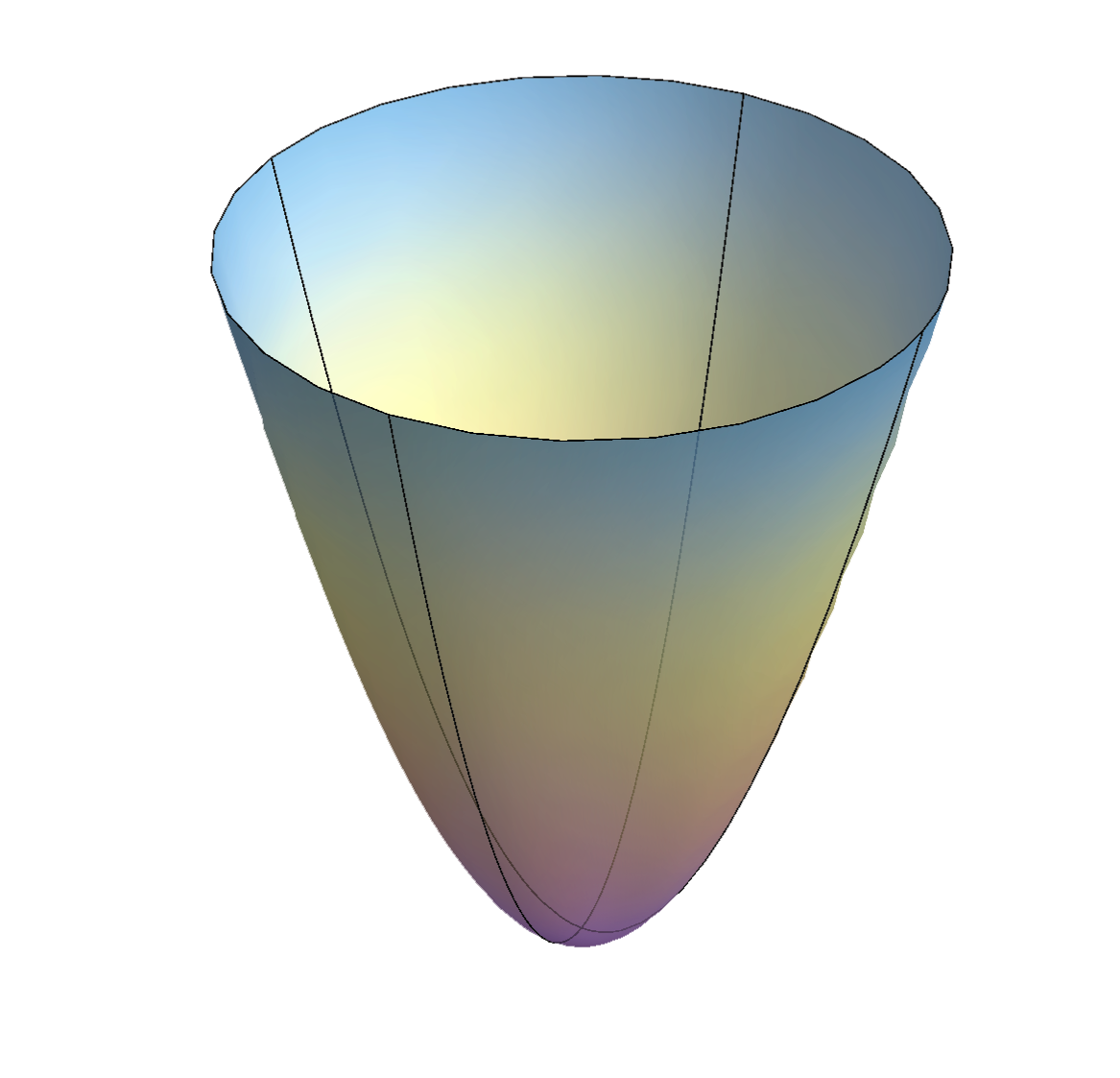right-click on the image $$\rightarrow$$ PLAY
 HYPERBOLIC PARABOLOID
 $$\frac{x^2}{a^2}-\frac{y^2}{b^2}=2cz$$ Doubly ruled surface - through every point on the surfaces there are two lines on the surfaces, two systems of rulings. Real plane intersections are: the parabolas, the hyperbolas and degenerated conics - pairs of real lines (intersection with the tangent planes).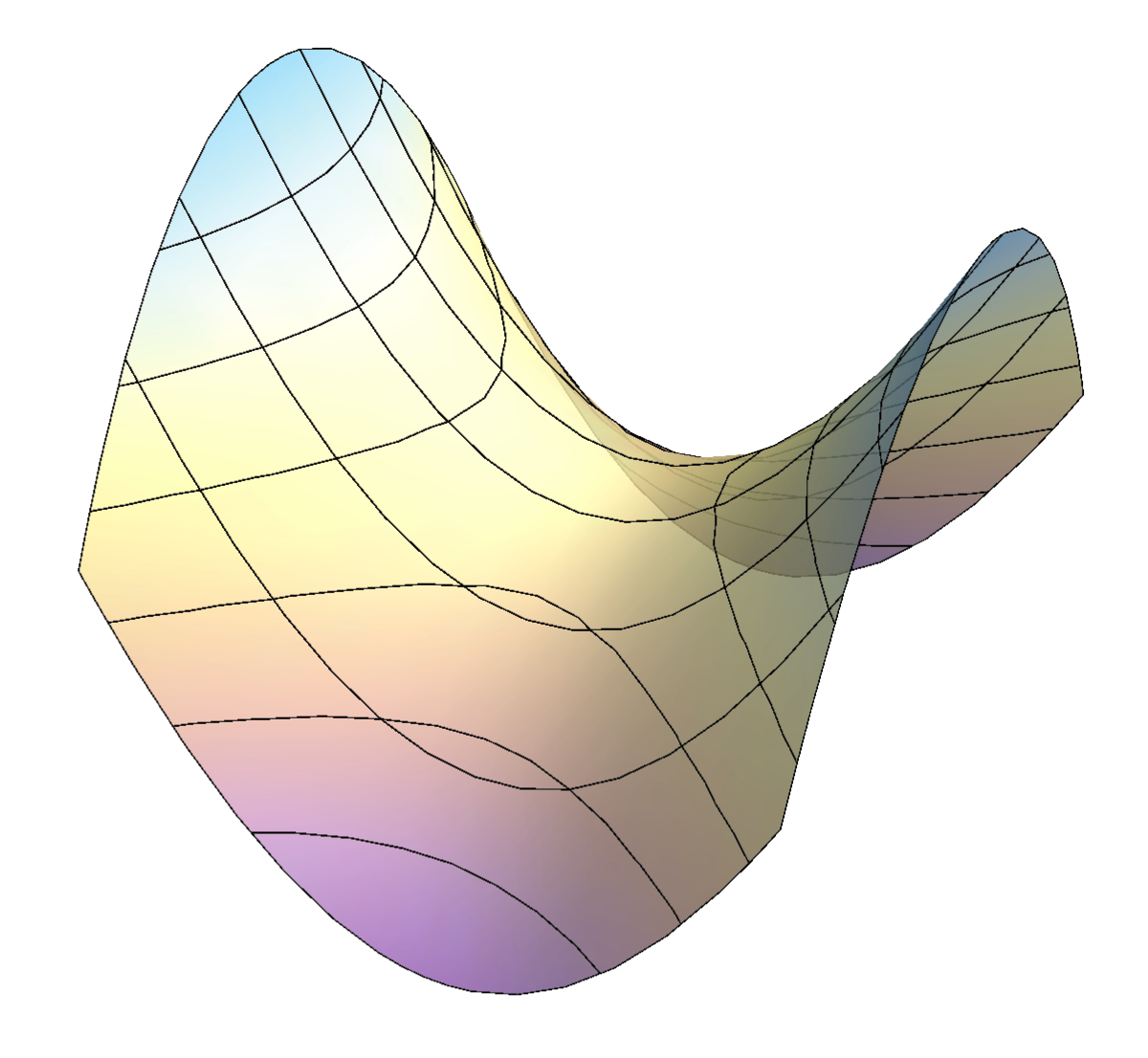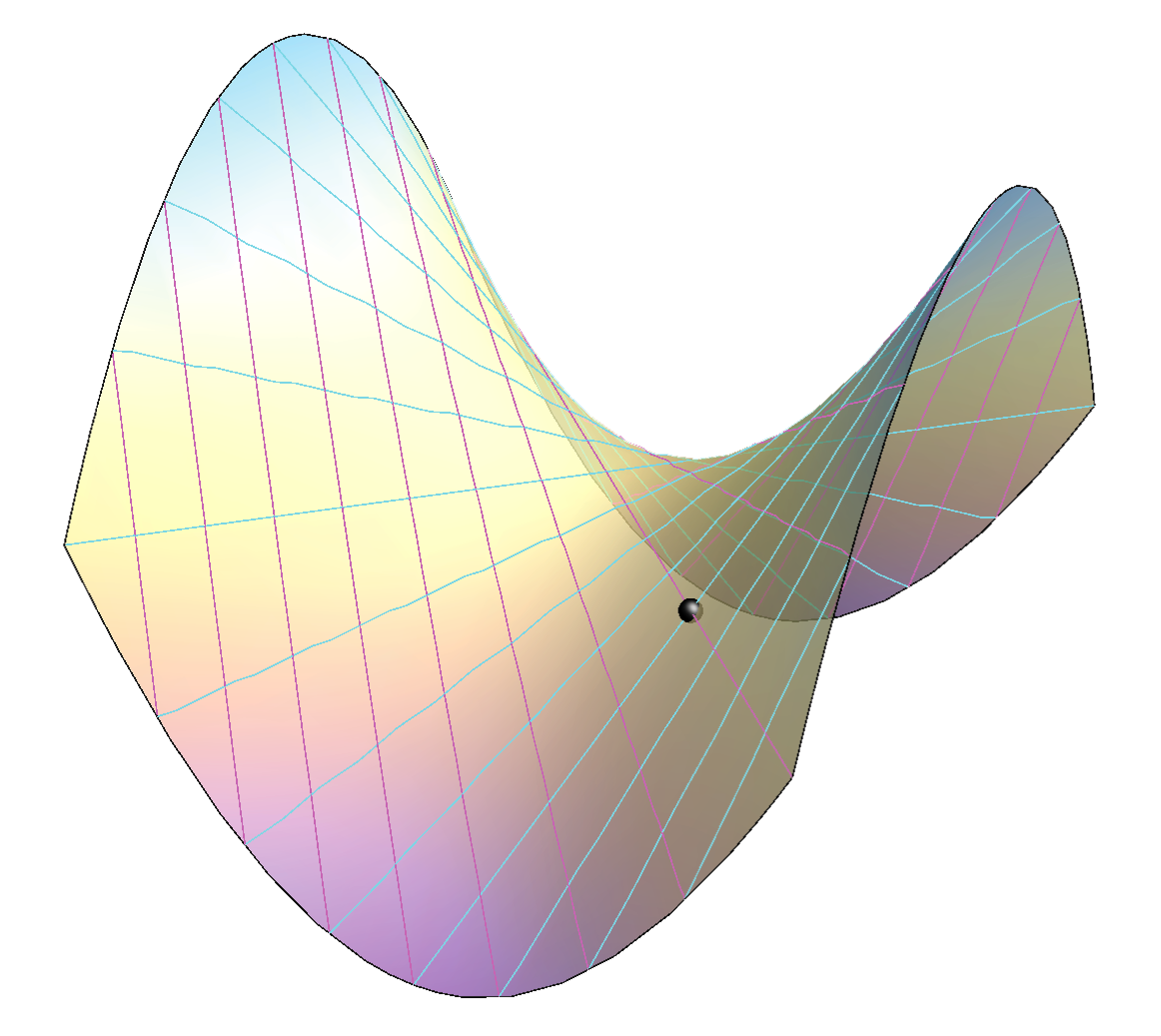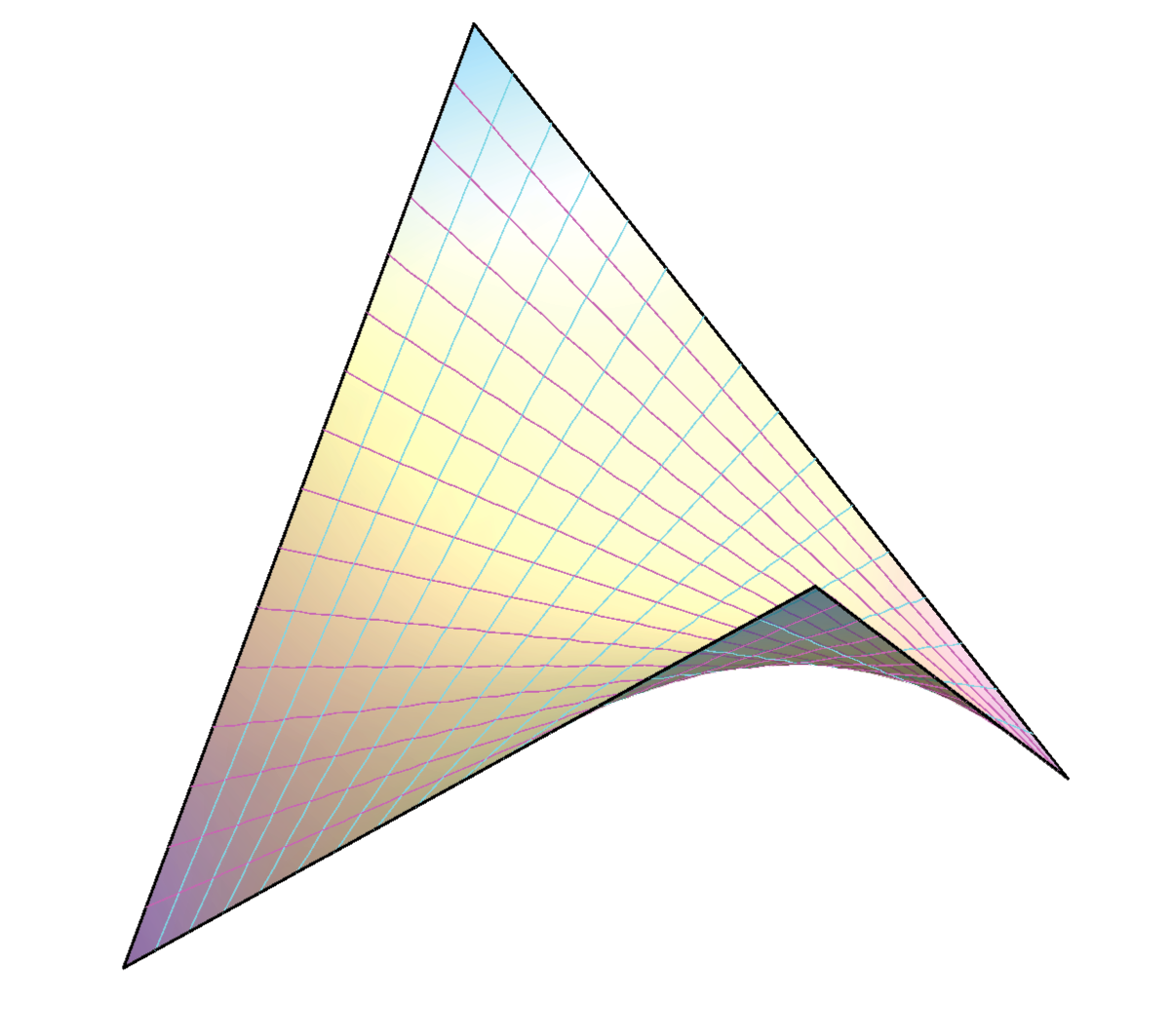Created by Sonja Gorjanc, translated by Helena Halas and Iva Kodrnja - 3DGeomTeh - Developing project of the University of Zagreb